MM Estimation

MM estimation is a combination of high-breakdown-value estimation and efficient estimation, which was introduced by Yohai (1987). It has the following three steps:

1. Compute an initial (consistent) high-breakdown-value estimate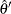. The ROBUSTREG procedure provides two kinds of estimates as the initial estimate: the LTS estimate and the S estimate. By default, the LTS estimate is used because of its speed and high breakdown value. The breakdown value of the final MM estimate is decided by the breakdown value of the initial LTS estimate and the constant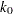in the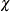function. To use the S estimate as the initial estimate, you specify the INITEST=S option in the PROC statement. In this case, the breakdown value of the final MM estimate is decided only by the constant. Instead of computing the LTS estimate or the S estimate as the initial estimate, you can also specify the initial estimate explicitly by using the INEST= option in the PROC statement. See the section INEST= Data Set for details.

2. Find
such that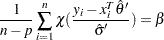where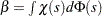.

The ROBUSTREG procedure provides two choices for: Tukey’s bisquare function and Yohai’s optimal function.

Tukey’s bisquare function, which you can specify with the option CHIF=TUKEY, is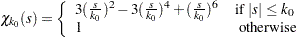wherecan be specified with the K0= option. The defaultis 2.9366 such that the asymptotically consistent scale estimatehas the breakdown value of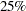.

Yohai’s optimal function, which you can specify with the option CHIF=YOHAI, is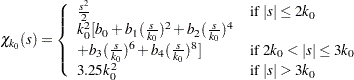where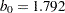,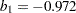,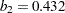,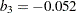, and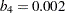. You can specifywith the K0= option. The defaultis 0.7405 such that the asymptotically consistent scale estimatehas the breakdown value of.

3. Find a local minimum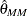of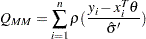such that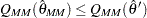. The algorithm for M estimation is used here.

The ROBUSTREG procedure provides two choices for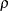: Tukey’s bisquare function and Yohai’s optimal function.

Tukey’s bisquare function, which you can specify with the option CHIF=TUKEY, is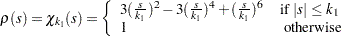where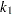can be specified with the K1= option. The defaultis 3.440 such that the MM estimate has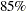asymptotic efficiency with the Gaussian distribution.

Yohai’s optimal function, which you can specify with the option CHIF=YOHAI, is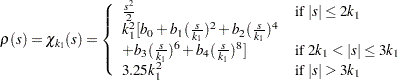wherecan be specified with the K1= option. The defaultis 0.868 such that the MM estimate hasasymptotic efficiency with the Gaussian distribution.

### Algorithm

The initial LTS estimate is computed using the algorithm described in the section LTS Estimate. You can control the quantile of the LTS estimate with the option INITH=h, where h is an integer between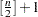and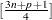. By default,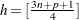, which corresponds to a breakdown value of around.

The initial S estimate is computed using the algorithm described in the section S Estimate. You can control the breakdown value and efficiency of this initial S estimate by the constant, which can be specified with the K0 option.

The scale parameter
is solved by an iterative algorithm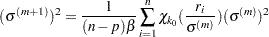where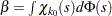.

Once the scale parameter is computed, the iteratively reweighted least squares (IRLS) algorithm with fixed scale parameter is used to compute the final MM estimate.

### Convergence Criteria

In the iterative algorithm for the scale parameter, the relative change of the scale parameter controls the convergence.

In the iteratively reweighted least squares algorithm, the same convergence criteria for the M estimate used before are used here.

### Bias Test

Although the final MM estimate inherits the high-breakdown-value property, its bias due to the distortion of the outliers can be high. Yohai, Stahel, and Zamar (1991) introduced a bias test. The ROBUSTREG procedure implements this test when you specify the BIASTEST option in the PROC statement. This test is based on the initial scale estimateand the final scale estimate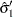, which is the solution of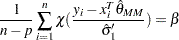Let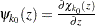and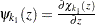. Compute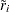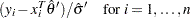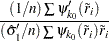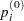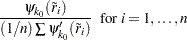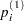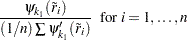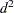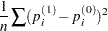Let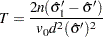Standard asymptotic theory shows that T approximately follows a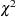distribution with p degrees of freedom. If T exceeds thequantile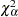of thedistribution with p degrees of freedom, then the ROBUSTREG procedure gives a warning and recommends that you use other methods. Otherwise, the final MM estimate and the initial scale estimate are reported. You can specifywith the ALPHA= option following the BIASTEST option. By default, ALPHA=0.99.

### Asymptotic Covariance and Confidence Intervals

Since the MM estimate is computed as a M estimate with a known scale in the last step, the asymptotic covariance for the M estimate can be used here for the asymptotic covariance of the MM estimate. Besides the three estimators H1, H2, and H3 as described in the section Asymptotic Covariance and Confidence Intervals, a weighted covariance estimator H4 is available. H4 is calculated as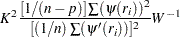where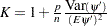is the correction factor and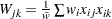,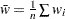.

You can specify these estimators with the option ASYMPCOV= [H1 | H2 | H3 | H4]. The ROBUSTREG procedure uses H4 as the default. Confidence intervals for estimated parameters are computed from the diagonal elements of the estimated asymptotic covariance matrix.

### R Square and Deviance

The robust version of R-square for the MM estimate is defined as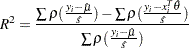and the robust deviance is defined as the optimal value of the objective function on the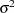scale,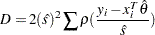where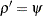,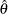is the MM estimator of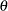,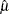is the MM estimator of location, andis the MM estimator of the scale parameter in the full model.

### Linear Tests

For MM estimation, the sametest andtest used for M estimation can be used. See the section Linear Tests for details.

### Model Selection

For MM estimation, the same two model selection methods used for M estimation can be used. See the section Model Selection for details.